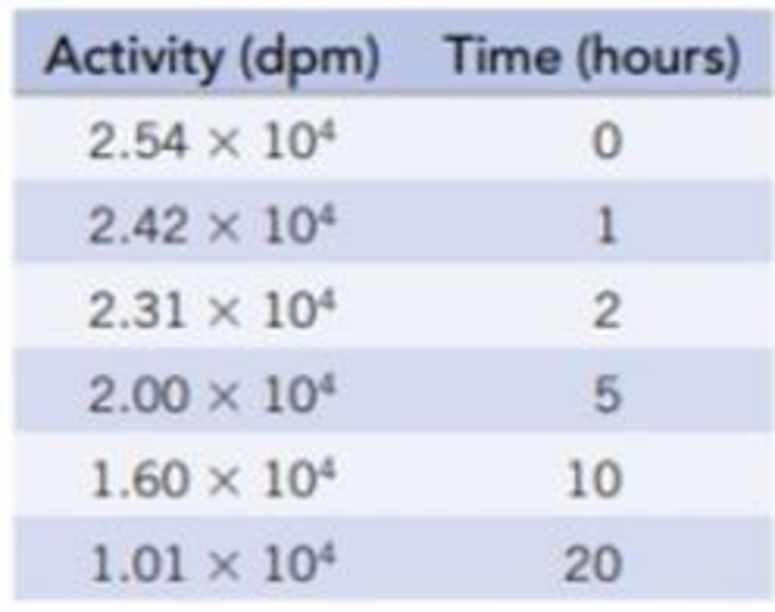Chapter 25, Problem 68IL

Chapter
Section
Textbook Problem

Sodium-23 (in a sample of NaCI) is subjected to neutron bombardment in a nuclear reactor to produce 24Na. When removed from the reactor, the sample is radioactive, with β activity of 2.54 × 104 dpm. The decrease in radioactivity over time was studied, producing the following data:(a) Write equations for the neutron capture reaction and for the reaction in which the product of this reaction decays by β emission.(b) Determine the half-life of sodium-24.

(a)

Interpretation Introduction

Interpretation:

An equation for the neutron capture reaction and for the reaction in which the product of this reaction decays by beta-emission has to be written.

Concept introduction:

Balancing nuclear reaction equation: The balanced nuclear reaction should conserve both mass number and atomic number.

• The sum of the mass numbers of the reactants should be equal to the sum of mass numbers of the products in the reaction.
• The sum of atomic numbers (or the atomic charge) of the reactants should be equal to the sum of atomic numbers (or the atomic charge) of the products in the reaction.
Explanation

The neutron capture of sodium-23 produces sodium-24. Then again sodium-24 transmuted to magnesium-24 by the emission of beta particle. On writing the equations, the sum of atomic and mass number must be equal.

N1123a+n01N

(b)

Interpretation Introduction

Interpretation:

Half-life of Sodium-23 has to be determined.

Concept introduction:

Concept Introduction:

Radiocarbon dating: The dynamic equilibrium exists in all living organism by exhaling or inhaling, maintain the same ratio of 12C and 14C that takes in. when the living organism dies, the intake of 14C stops and it’s the ratio no longer exhibits equilibrium; undergoes radioactive decay.

Half-life period: The time required to reduce to half of its initial value.

Formula used to calculate half-life:

t1/2=0.693kwhere,kis rate constant.(or)ln[N]t- ln[N]0= -kt

Still sussing out bartleby?

Check out a sample textbook solution.

See a sample solution

The Solution to Your Study Problems

Bartleby provides explanations to thousands of textbook problems written by our experts, many with advanced degrees!

Get Started

Calculate your protein RDA.

Understanding Nutrition (MindTap Course List)

What is a life cycle?

Biology: The Dynamic Science (MindTap Course List)

Define the following terms: a. chromosome b. chromatin

Human Heredity: Principles and Issues (MindTap Course List)